# Energy

Student Learning Objectives
Lessons / Lecture Notes
Important Equations
Example Problems
Applets and Animations

Student Learning Objectives

• To explicitly use systems-based thinking to develop a model of energy—what it is, how it is transformed, and how it is transferred.
• To introduce the concepts of work and kinetic energy.
• To learn about the relationship between work and kinetic energy through the work-kinetic energy theorem.
• To introduce a model of thermal energy and dissipative forces.
• To differentiate between conservative and non-conservative forces.
• To learn Hooke’s law for springs and the new idea of a restoring force.
• To expand the basic energy model by introducing potential energy.
• To learn to use and interpret energy bar charts and energy diagrams.
• To develop a complete statement of the energy principle and to apply the energy principle to isolated systems in which energy is conserved.
• To understand and use the concepts of kinetic, potential, and thermal energy.
• To solve problems using the law of conservation of energy.

Lessons / Lecture Notes

The Physics Classroom (conceptual)

PY105 Notes from Boston University (algebra-based):

Physics 2A notes from Dr. Bobby W.S. Lau (algebra-based)

HyperPhysics (calculus-based)

Important Equations (for algebra-based Physics)

Example Problems

Example Problems for algebra-based physics (from College Physics 2nd Edition by Knight, Jones, and Field):

Example Problems (Energy and Work)
Solutions to Example Problems (Energy and Work)

Example Problems for calculus-based physics (from Physics for Scientists and Engineers 4th Edition by Knight):

Example Problems (Work and Kinetic Energy) | Example Problems (Interactions and Potential Energy)
Solutions to Example Problems (Work and Kinetic Energy) | Solutions to Example Problems (Interactions and Potential Energy)

Applets and Animations
 Work and Kinetic Energy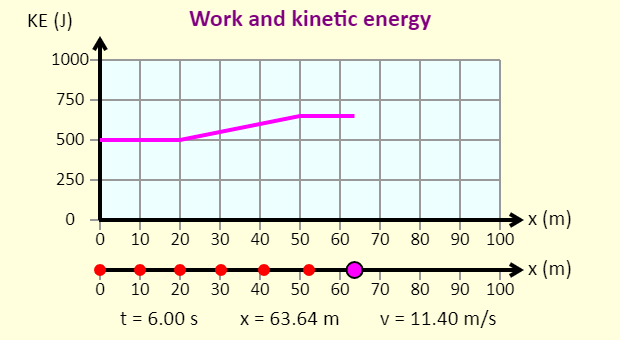This simulation is similar to the impulse/momentum simulation, but in this one the force is applied as the object moves through a specific distance, as opposed to the force being applied for a specific amount of time. When a constant force is applied over a particular distance, the momentum vs. position graph would be non-linear, but the kinetic energy vs. position graph would be linear, so that's the graph we plot here. Just for fun, you can see the momentum vs. position graph, too, if you'd like to. Energy Bar Graphs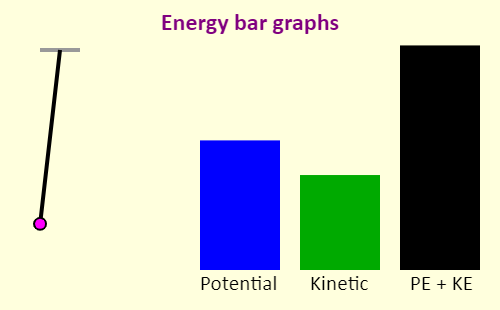This simulations shows the kinetic and potential energies of a swinging pendulum. Sliding Down a Ramp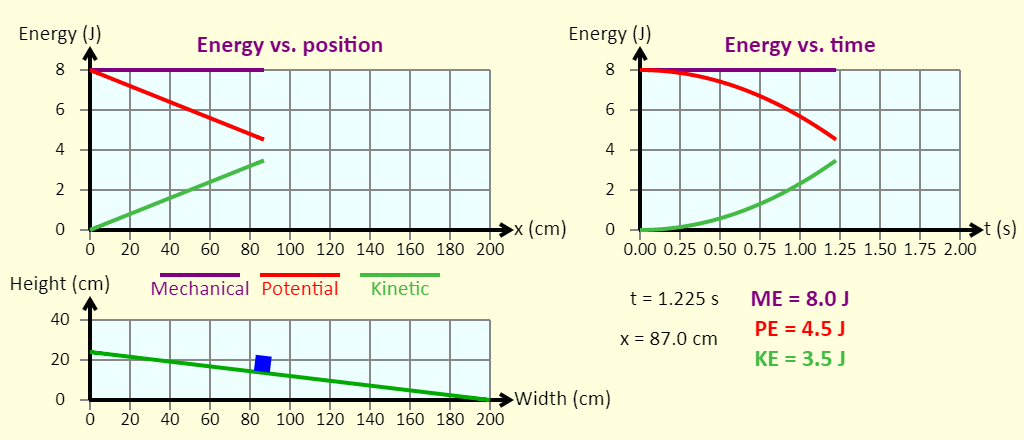The object slides down the ramp. You can plot the total mechanical energy (purple), gravitational potential energy (red), kinetic energy (green), and the thermal energy (black) as a function of time or position. Sliding Down a Ramp (with Spring)The object slides down the ramp without friction, toward a spring at the bottom. You can plot the gravitational potential energy (red), the kinetic energy (green), and the elastic potential energy (blue) as a function of time or position. The Ramp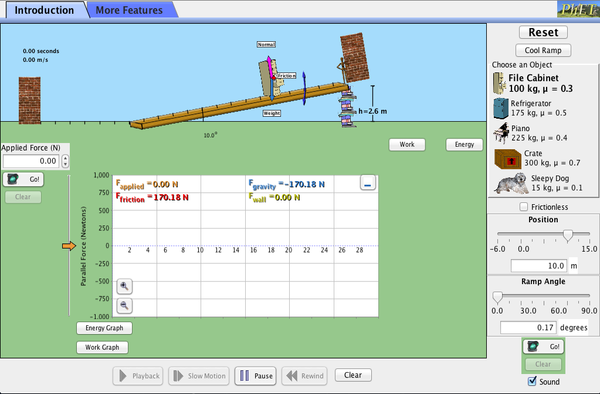Explore forces, energy and work as you push household objects up and down a ramp. Lower and raise the ramp to see how the angle of inclination affects the parallel forces acting on the file cabinet. Graphs show forces, energy and work Energy Skate Park: BasicsLearn about conservation of energy with a skater gal! Explore different tracks and view the kinetic energy, potential energy and friction as she moves. Build your own tracks, ramps, and jumps for the skater. Energy Skate Park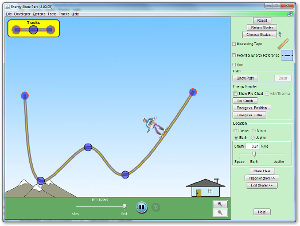Learn about conservation of energy with a skater dude! Build tracks, ramps and jumps for the skater and view the kinetic energy, potential energy and friction as he moves. You can also take the skater to different planets or even space! CoasterAnimation shows a car coasting on a curve. Losses of energy due to frictional forces have been ignored in modeling the behavior of the car. Besides energy conservation ideas, some dynamics of circular motion ideas also get highlighted. The car starts with negligible velocity from the initial position. The dots on the path should help to relate to changes in the velocity of the car as it moves along the path. Conservation of Energy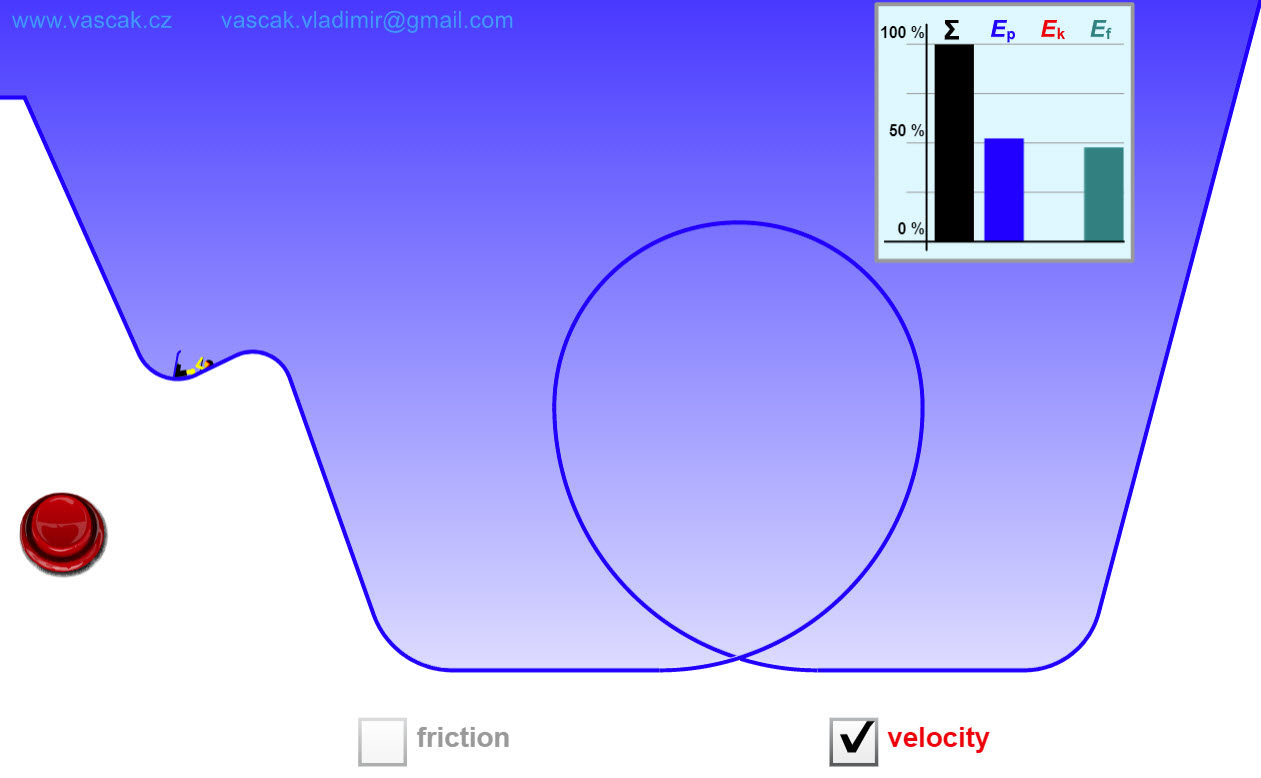This fun applet lets you adjust the initial conditions of a skier to investigate conservation of energy. Energy Forms and ChangesExplore how heating and cooling iron, brick, water, and olive oil adds or removes energy. See how energy is transferred between objects. Build your own system, with energy sources, changers, and users. Track and visualize how energy flows and changes through your system.